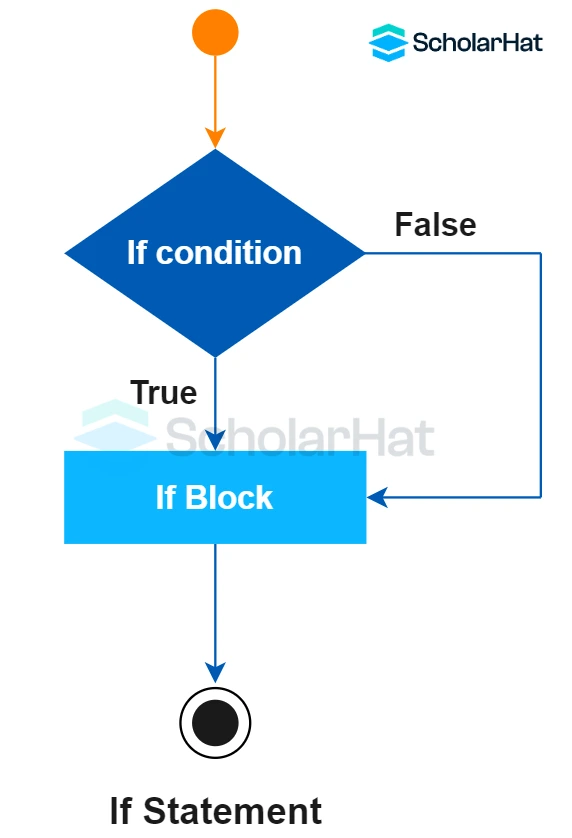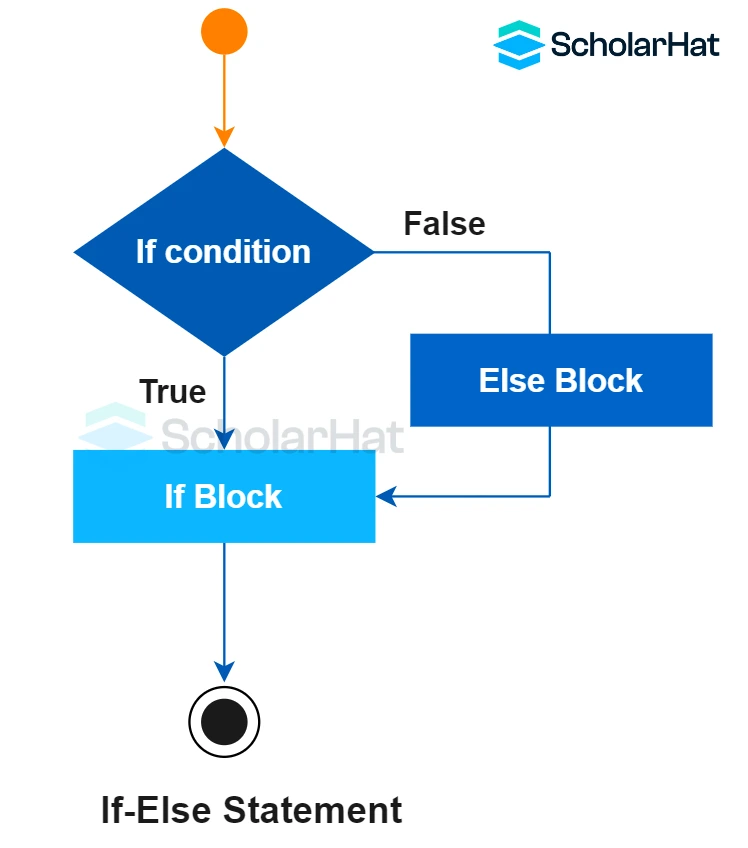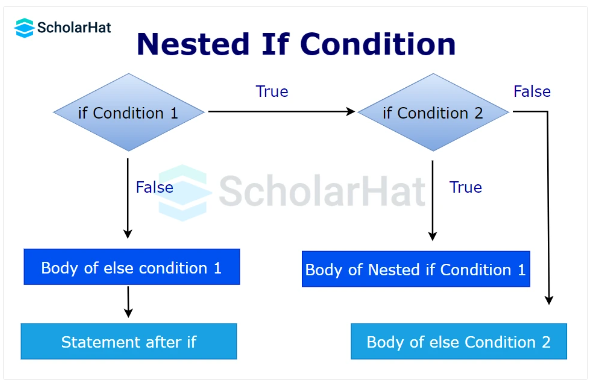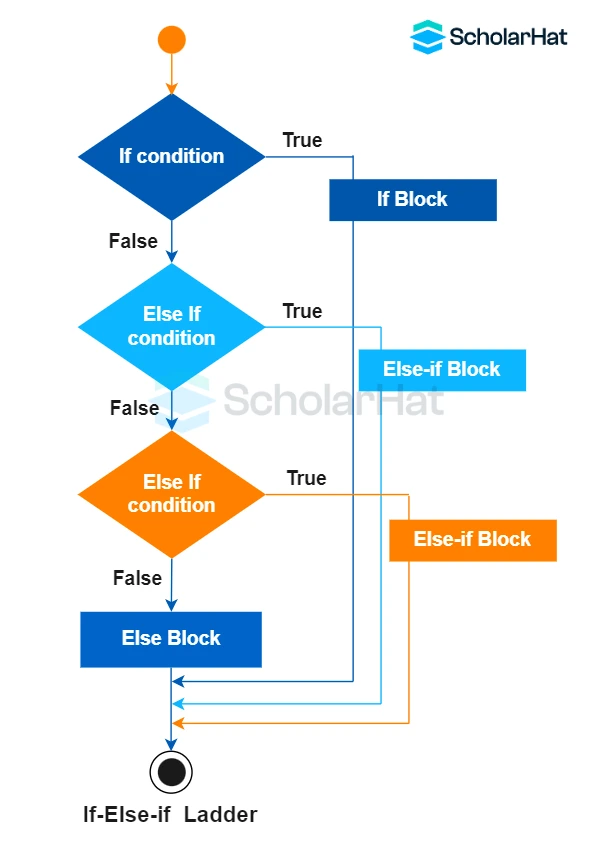# Conditional Statements in C++: if , if..else, if-else-if and nested if

24 Nov 2023
Beginner
3.79K ViewsLearn via Video Course & by Doing Hands-on Labs

## Conditional Statements in C++: An Overview

The flow of a program is managed using conditional statements in C++. If a condition is true inside the `if`statement, the body of the statement executes. If false, `else`is used to specify an alternate course of action. You can check several criteria sequentially using the `else-if`statement and learn C++ from scratch. For multi-way branching based on the result of an expression, use the `switch` statement. You can also check our C++ Certification Course for in-depth knowledge of C++

## Conditional Statements in C++ programming?

In C++, conditional statements are control structures that let code make decisions. These statements are also known as decision-making statements. They are of the type `if` statement, `if-else`, `if else-if ladder`, `switch`, etc. These statements determine the flow of the program execution.

## Types of Conditional Statements in C++

In this article, we'll discuss the first kind of conditional statement i.e. `if...else` statements in C++. We'll discuss the `switch` statement in the next tutorial switch statement in C++.

## if … else statements

`if-else` is the first kind of control statement in C++. In this, the operations are performed according to some specified condition. The statements inside the body of the `if` block get executed if and only if the given condition is true.

## Variants of if-else statements in C++

We have four variants of `if-else` statements in C++. They are:

1. `if` statement in C++
2. `if-else` statement in C++
3. `if else-if ladder` in C++
4. `nested if` statement in C++

### if statement in C++

`if` statements in C++ are one of the most simple statements for deciding in a program. When you want to print any code only upon the satisfaction of a given condition, go with the simple `if` statement.#### Syntax

``````if(testcondition)
{
// Statements to execute if
// condition is true
}
``````

• In the `if` statement, the test condition is in `()`.
• If the `testcondition` becomes `true`, the body of the `if` block executes else no statement of the `if` block gets printed.

#### Example

``````// C++ program to understand if statement
#include <iostream>
using namespace std;
int main()
{
int i = 11;
if (i > 15)
{
cout << "11 is greater than 15";
}
cout << "I am Not in if";
return 0;
}``````

This C++ code initializes the integer `i`to 11 and then checks to see if it is greater than 15 using an `if`statement. It outputs "I am Not in if" to the console because 11 is not greater than 15.

#### Output

``````I am Not in if
``````

### if-else statement in C++

It is an extension of the `if` statement. Here, there is an `if` block and an `else` block. When one wants to print output for both cases - `true` and `false`, use the `if-else` statement.#### Syntax

``````if (testcondition)
{
// Executes this block if
// condition is true
}
else
{
// Executes this block if
// condition is false
}
``````

#### Example

``````// C++ program to understand the if-else statement
#include <iostream>
using namespace std;
int main()
{
int A = 16;
if (A < 15)
cout << "A is smaller than 15";
else
cout << "A is greater than 15";
return 0;
}
``````

This C++ program initializes the integer variable `A`to 16 and then checks to see if it is less than 15 using the `if-else`statement. It outputs "A is greater than 15" to the console because it isn't.

#### Output

``````A is greater than 15
``````

### nested if statement in C++

We can include an `if-else` block in the body of another `if-else` block. This becomes a `nested if-else` statement.#### Syntax

``````if(test condition1)
{ //if condition1 becomes true
if(test condition1.1)
{
//code executes if both condition1 and condition 1.1 becomes true
}
else
{
//code executes if condition1 is true but condition 1.1 is false
}
}
else
{
//code executes if condition1 becomes false
}
``````

#### Example

``````// C++ program to understand nested-if statement
#include <iostream>
using namespace std;
int main() {
int a = 10;
int b = 5;
if (a > b) {
// Control goes to the nested if
if (a % 2 == 0) {
cout << a << " is even";
}
else {
cout << a << " is odd";
}
}
else {
cout << a << " is not greater than " << b;
}
return 0;
}
``````

• In the above code, the first `if` statement checks whether `a>b`.
• If `a` is greater than `b`, the `nested if` statement is checked.
• If the `nested if` condition is `false`, the `else` statement in the `nested if` block gets executed.
• If the first `if` statement evaluates to `false`, the `nested if` block gets skipped and the `else` block executes.

#### Output

``````10 is even
``````

### if else-if ladder in C++

It is an extension of the `if-else` statement. If we want to check multiple conditions if the first `if`condition becomes `false`, use the `if else-if ladder`. If all the `if` conditions become `false` the `else` block gets executed.#### Syntax

``````if (testcondition1)
statement;
else if (testcondition2)
statement;
.
.
else
statement;
``````

#### Example

``````// C++ program to understand if-else-if ladder
#include <iostream>
using namespace std;
int main()
{
int A = 20;
if (A == 10)
cout << "A is 10";
else if (A == 15)
cout << "A is 15";
else if (A == 20)
cout << "A is 20";
else
cout << "A is not present";
return 0;
}``````

The`if else-if`ladder is shown in this section of C++ code. It sequentially compares the value of `A`to several conditions. It outputs "A is 20"as the condition matched and comes out of the ladder.

#### Output

``A is 20``
##### Summary

This article gives a vast idea about `if-else` conditional statement in C++ language, including its various types with syntax and examples. If you've understood everything we went over in this blog post, congratulations! Now you can check out for a C++ certificationto get some more insights and practice on such an important programming concept.

Share Article
Similar Articles